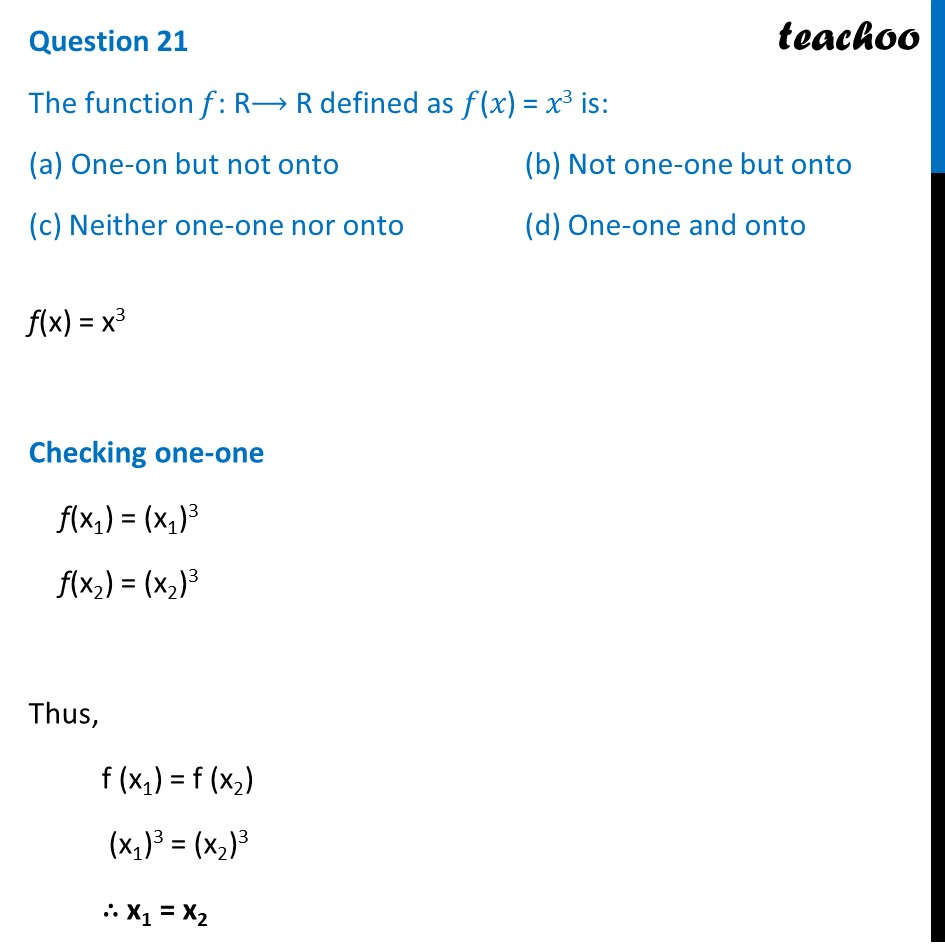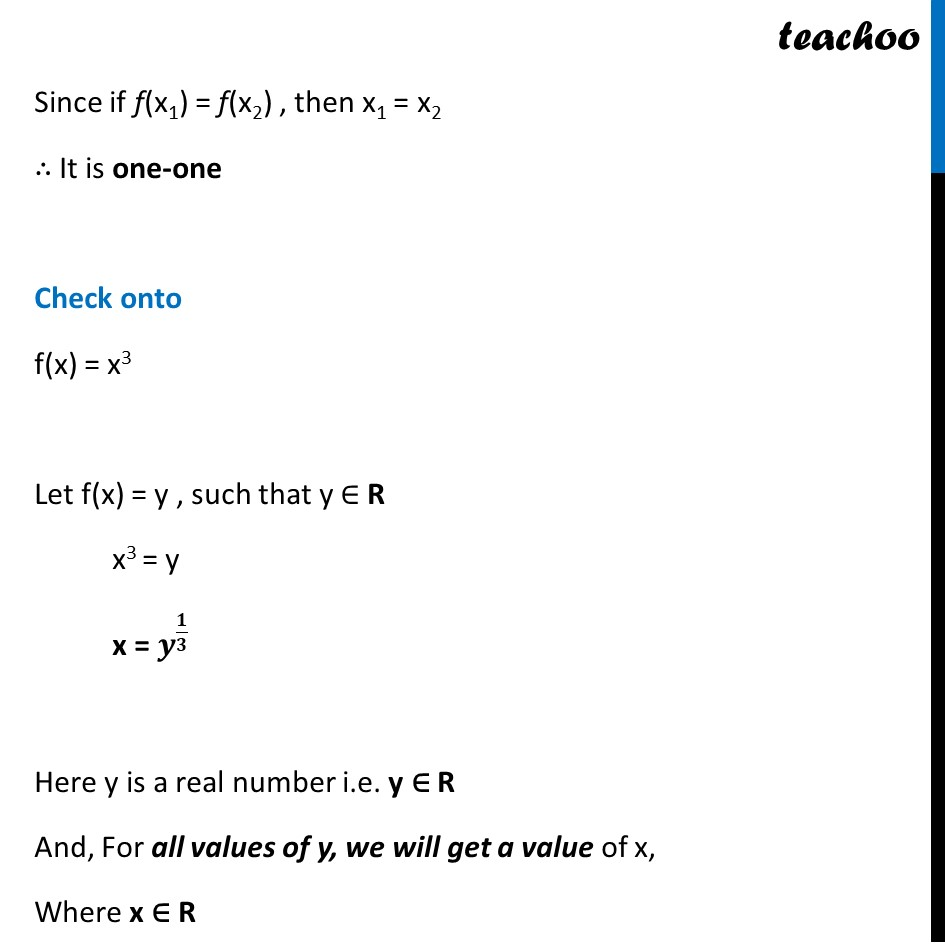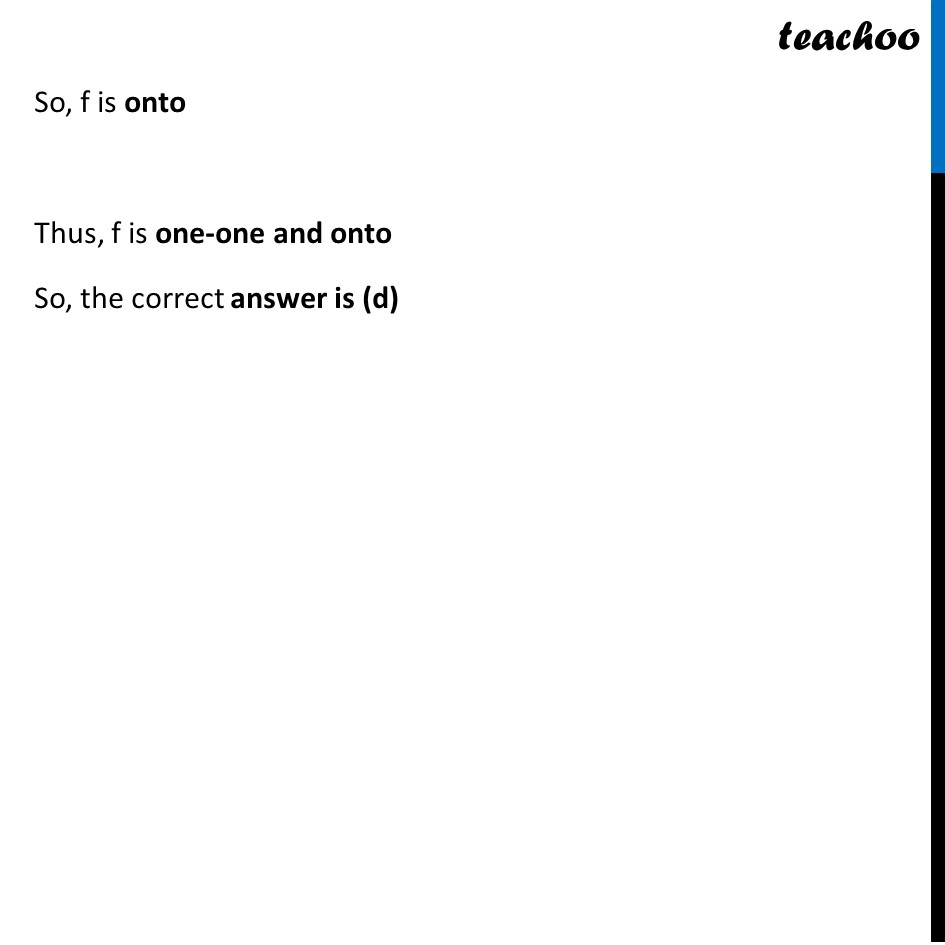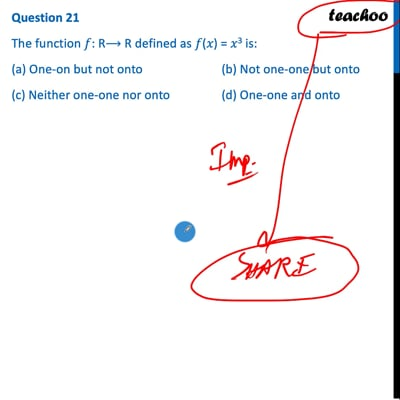CBSE Class 12 Sample Paper for 2022 Boards (MCQ Based - for Term 1)

Class 12
Solutions of Sample Papers and Past Year Papers - for Class 12 Boards

## (a) One-on but not onto  (b) Not one-one but onto (c) Neither one-one nor onto  (d) One-one and onto

This question is inspired from Question 1 (Choice 1) - CBSE Class 12 Sample Paper for 2021 BoardsThis video is only available for Teachoo black users

Introducing your new favourite teacher - Teachoo Black, at only ₹83 per month

### Transcript

Question 21 The function 𝑓: R⟶ R defined as 𝑓(𝑥) = 𝑥3 is: (a) One-on but not onto (b) Not one-one but onto (c) Neither one-one nor onto (d) One-one and onto f(x) = x3 Checking one-one f(x1) = (x1)3 f(x2) = (x2)3 Thus, f (x1) = f (x2) (x1)3 = (x2)3 ∴ x1 = x2 Since if f(x1) = f(x2) , then x1 = x2 ∴ It is one-one Check onto f(x) = x3 Let f(x) = y , such that y ∈ R x3 = y x = 𝒚^(𝟏/𝟑) Here y is a real number i.e. y ∈ R And, For all values of y, we will get a value of x, Where x ∈ R So, f is onto Thus, f is one-one and onto So, the correct answer is (d)